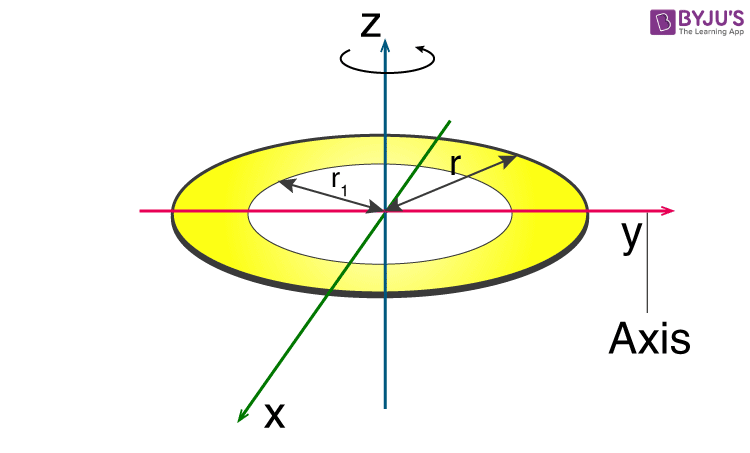Checkout JEE MAINS 2022 Question Paper Analysis : Checkout JEE MAINS 2022 Question Paper Analysis :

# Moment Of Inertia Of Annular Disc

Moment of inertia of an annular disc that is uniform and having mass (m), thickness (t), inner radius (R1) and outer radius (R2) is expressed as;

 I = ½ M (R22 + R12 )

Here, we have to consider that the disc is rotating about an axis passing through the centre.

## Derivation Of Moment Of Inertia Of Annular Disc1. We will start by recalling the moment of inertia expression which is given as;

dI = r2 dm

In this, we can consider dm as the mass of volume dV. We will then first consider the moment of inertia about the z-axis and we get the expression;

Izz = OR r2dm

Like the relation in a disc, the mass dm of the volume element dV is related to volume and density by;

dm = ρ dV

We then have to calculate dV. Here we will assume that the disc will have a uniform density. Here we will also consider a ring to be at radius r with width dr and thickness t. We will get;

dV = (2 π r dr)t

If we substitute the values for dm we get;

dm = ρ (2 πt ) r dr

2. We can then write a new expression for Izz. It will be;

Izz = R1R2 r2dm

Izz = 2 π ρ t R1R2 r3dr

Now we integrate between R1 and R2. We will now have;

Izz = 2 π  ρ t [R24/4 – R14 / 4]

Izz = (½) π ρ t [ R24 – R14] ……………(Equation 1)

However, we can also express it as [ R22 + R12][ R22 – R12]. Hence, the equation now becomes;

Izz = ½ πρt [ R22 + R12][ R22 – R12] ……………..(Equation 2)

3. Now we need to find the total mass of the annular disk. We will consider the total mass (M2) of a disc of radius R2 and subtract it from the mass (M1) of a disc of radius R1.

Mass = Density x Area x Thickness

We will get,

M2 = π ρ t R22

M1 = π ρ t R12

So the mass of the annular disc is given as;

Ma = M2 – M1

Ma = π ρ t R22 – πpt R12

Ma = π ρ t (R22 – R12)

4. In the next step, we will substitute the above values in equation 2, which is.

Izz = ½ π ρ t [ R22 + R12][ R22 – R12]

Once the values are added and after integration, we can now write the expression for moment of inertia of an annular disc as;

Izz = ½ Ma (R22 + R12)

### Parallel Axis theorem## Frequently Asked Questions on Moment of Inertia of Annular Disc

### What is the moment of inertia of an annular disc about its central axis?

The moment of inertia of an annular disc of mass M with outer and inner radii R and r is given by
Ic = M(R2 + r2)/2

### What is moment of inertia?

The moment of inertia decides the amount of torque needed for a desired angular acceleration or a property that resists angular acceleration when measured in relation to the rotation axis.

### What is the formula for the moment of inertia?

Moment of inertia
I = miri2
The moment of inertia is given by the product of the mass (m) of the particle and the square of the distance between each particle from the axis of rotation (r)